Most Affordable JEE | NEET | 8,9,10 Preparation by Kota's Top IITian Doctor Faculties

# States of Matter - JEE Main Previous Year Questions with SolutionsJEE Main Previous Year Papers Questions of Chemistry With Solutions are available at eSaral. Simulator   Previous Years AIEEE/JEE Mains Questions
Q. The molecular velocity of any gas is :- (1) inversely proportional to the square root of temperature (2) inversely proportional to absolute temperature (3) directly proportional to square of temperature (4) directly proportional to square root of temperature [AIEEE-2011]
Ans. (4)
Q. , v and u represent most probable velocity, average velocity and root mean square velocity respectively of a gas at a particular temperature. The correct order among the following is - (1)  > u > v (2) v > u >  (3) u > v >  (4) u >  > v [JEE(Main)-2012]
Ans. (3)
Q. An open vessel at 300 K is heated till $\frac{2}{5}$th of the air in it is expelled. Assuming that the volume of the vessel remains constant, the temperature to which the vessel is heated is :- (1) 750 K (2) 400 K (3) 500 K (4) 1500K [JEE(Main-online)-2012]
Ans. (3)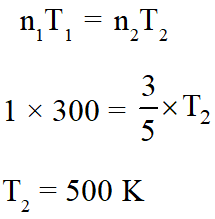Q. For 1 mol of an ideal gas at constant temperature T, the plot of (log P) against(logV) is a (P : Pressure, V : Volume) :- (1) Straight line parallel to x-axis (2) Curve starting at origin] (3) Straight line with a negative slope (4) Straight line passing through origin [JEE(Main-online)-2012]
Ans. (3) PV = nRT log (PV) = log (nRT) log P + log V = log (nRT) log P = –log V + log (nRT) y = – mx + C slope $=\tan \theta=-1$
Q. The relationship among most probable velocity, average velocity and root mean square velocity is respectively :- (1) $\sqrt{2}: \sqrt{8 / \pi}: \sqrt{3}$ (2) $\sqrt{2}: \sqrt{3}: \sqrt{8 / \pi}$ (3) $\sqrt{3}: \sqrt{8 / \pi}: \sqrt{2}$ (4) $\sqrt{8 / \pi}: \sqrt{3}: \sqrt{2}$ [JEE(Main-online)-2012]
Ans. (1)
Q. Which one of the following is the wrong assumption of kinetic theory of gases ? (1) All the molecules move in straight line between collision and with same velocity. (2) Molecules are separated by great distances compared to their sizes. (3) Pressure is the result of elastic collision of molecules with the container's wall. (4) Momentum and energy always remain conserved. [JEE(Main-online)-2013]
Ans. (1)
Q. By how many folds the temperature of a gas would increase when the root mean square velocity of the gas molecules in a container of fixed volume is increased from $5 \times 10^{4} \mathrm{cm} /$ s to $10 \times 10^{4} \mathrm{cm} / \mathrm{s} ?$ (1) Four (2) three (3) Two (4) Six [JEE(Main-online)-2013]
Ans. (1) $\mathrm{u}_{\mathrm{rms}} \propto \sqrt{\mathrm{T}}$ $\left(\frac{5 \times 10^{4}}{10 \times 10^{4}}\right)=\frac{\mathrm{T}_{1}}{\mathrm{T}_{2}}$ $\frac{1}{4}=\frac{T_{1}}{T_{2}}$ $\mathrm{T}_{2}=4 \mathrm{T}_{1}$
Q. For gaseous state, if most probable speed is denoted by C, average speed by $\overline{\mathrm{c}}$ and mean square speed by C, then for a large number of molecules the ratios of these speeds are :- (1) $\mathrm{C}: \overline{\mathrm{C}}: \mathrm{C}=1.225: 1.128: 1$ (2) $\mathrm{C}: \overline{\mathrm{C}}: \mathrm{C}=1.128: 1.225: 1$ (3) $\mathrm{C}: \overline{\mathrm{C}}: \mathrm{C}=1: 1.128: 1.225$ (4) $\mathrm{C}: \overline{\mathrm{C}}: \mathrm{C}=1: 1.225: 1.128$ [JEE(Main-offline)-2013]
Ans. (3) $\mathrm{u}_{\mathrm{avg}}=\sqrt{\frac{8 \mathrm{RT}}{\pi \mathrm{M}}}$ $\mathrm{u}_{\mathrm{rms}}=\sqrt{\frac{3 \mathrm{RT}}{\mathrm{M}}}$ $\mathrm{u}_{\mathrm{mp}}=\sqrt{\frac{2 \mathrm{RT}}{\mathrm{M}}}$
Q. A gaseous compound of nitrogen and hydrogen contains 12.5%(by mass) of hydrogen. The density of the compound relative to hydrogen is 16. The molecular formula of the compound is : (1) $\mathrm{NH}_{2}$ (2) $\mathrm{NH}_{3}$ (3) $\mathrm{N}_{3} \mathrm{H}$ (4) $\mathrm{N}_{2} \mathrm{H}_{4}$ [JEE(Main-online)-2014]
Ans. (4)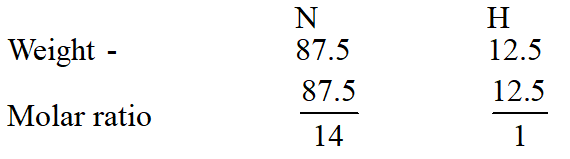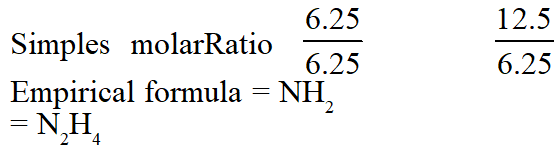Q. The initial volume of a gas cylinder is 750.0 mL. If the pressure of gas inside the cylinder changes from 840.0 mm Hg to 360.0 mm Hg, the final volume the gas will be (1)1.750 L (2) 7.50 L (3) 3.60 L (4) 4.032 L [JEE(Main-online)-2014]
Ans. (1) $\mathrm{P}_{1} \mathrm{V}_{1}=\mathrm{P}_{2} \mathrm{V}_{2}$ $840 \times 750=360 \times \mathrm{V}_{2}$ $\mathrm{V}_{2}=\frac{840 \times 750}{360}$
Q. The temperature at which oxygen molecules have the same root mean square speed as helium atoms have at 300 K is : (Atomic masses : He = 4 u, O = 16 u) (1) 1200 K (2) 600 K (3) 300 K (4) 2400 K [JEE(Main-online)-2014]
Ans. (4)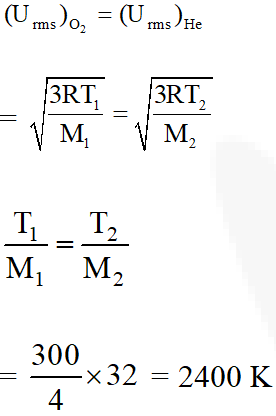Q. Two closed bulbs of equal volume(V) containing an ideal gas initially at pressure pi and temperature $\mathrm{T}_{1}$ are connected through a narrow tube of negligible volume as shown in the figure below. The temperature of one of the bulbs is then raised to $\mathrm{T}_{2}$. The final pressure $\mathrm{P}_{\mathrm{f}}$ is :-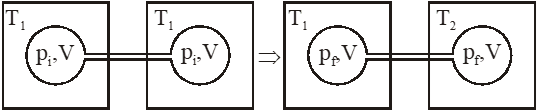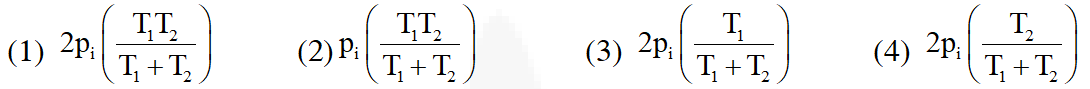[JEE(Main)-2016]
Ans. (4) Initial moles and final moles are equal $\left(\mathrm{n}_{\mathrm{T}}\right)_{\mathrm{i}}=\left(\mathrm{n}_{\mathrm{T}}\right)_{\mathrm{f}}$ $\frac{\mathrm{P}_{\mathrm{i}} \mathrm{V}}{\mathrm{RT}_{1}}+\frac{\mathrm{P}_{\mathrm{i}} \mathrm{V}}{\mathrm{RT}_{1}}=\frac{\mathrm{P}_{\mathrm{f}} \mathrm{V}}{\mathrm{RT}_{1}}+\frac{\mathrm{P}_{\mathrm{f}} \mathrm{V}}{\mathrm{RT}_{2}}$ $2 \frac{\mathrm{P}_{\mathrm{i}}}{\mathrm{T}_{\mathrm{i}}}=\frac{\mathrm{P}_{\mathrm{i}}}{\mathrm{T}_{\mathrm{i}}}+\frac{\mathrm{P}_{\mathrm{i}}}{\mathrm{T}_{2}}$ $\mathrm{P}_{\mathrm{f}}=\frac{2 \mathrm{P}_{\mathrm{i}} \mathrm{T}_{2}}{\mathrm{T}_{1}+\mathrm{T}_{2}}$
Q. 'a' and 'b' are Vander Waal's constants for gases. Chlorine is more easily liquefied than ethane because :-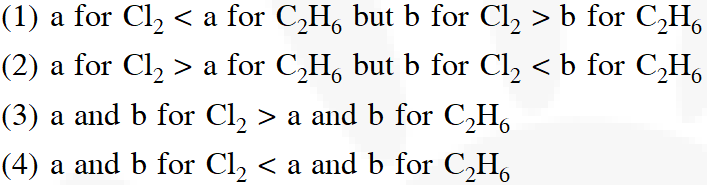[Aieee-2012]
Ans. (2) Higher the 'a' value, more easily the gas is liquified, lower the 'b' value, more easily the gas is liquified
Q. The compressibility factor for a real gas at high pressure is :- (1) $1-\frac{\text { Pb }}{\mathrm{RT}}$ (2) $1+\frac{\mathrm{RT}}{\mathrm{Pb}}$ (3) 1 (4) $1+\frac{\mathrm{Pb}}{\mathrm{RT}}$ [Aieee-2012]
Ans. (4) At high pressure, repulsion dominate. $\left(\mathrm{P}+\frac{\mathrm{a}}{\mathrm{Vm}^{2}}\right)(\mathrm{Vm}-\mathrm{b})=\mathrm{RT}$ P(Vm – b) = RT $\mathrm{So}, \mathrm{Z}=1+\frac{\mathrm{Pb}}{\mathrm{RT}}$
Q. If Z is the compressibility factor, van der Waals' equation at low pressure can be written as : $(1) Z=1-\frac{\mathrm{Pb}}{\mathrm{RT}}$ $(2) Z=1+\frac{\mathrm{Pb}}{\mathrm{RT}}$ (3) $\mathrm{Z}=1+\frac{\mathrm{RT}}{\mathrm{Pb}}$ (4) $\mathrm{Z}=1-\frac{\mathrm{a}}{\mathrm{V}_{\mathrm{m}} \mathrm{RT}}$ [JEE-MAINS-2014]
Ans. (4) At low pressure, attractions dominate. $\left(\mathrm{P}+\frac{\mathrm{a}}{\mathrm{Vm}^{2}}\right)(\mathrm{Vm}-\mathrm{b})=\mathrm{RT}$ $\left(\mathrm{P}+\frac{\mathrm{a}}{\mathrm{Vm}^{2}}\right)(\mathrm{Vm})=\mathrm{RT}$ $\mathrm{So}, \mathrm{Z}=1-\frac{\mathrm{a}}{\mathrm{VmRT}}$
Q. When does a gas deviate the most from it's ideal behaviour ? (1) At high pressure and low temperature (2) At high pressure and high temperature (3) At low pressure and low temperature (4) At low pressure and high temperature [JEEMAINS(online)-2015]
Ans. (1) Gas behaves most ideally at high T and low P while deviates most from ideal behaviour at high P and low T.

Yogesh
Oct. 4, 2023, 8:38 p.m.
Good for practice 🙏 sir
April 29, 2023, 6:35 a.m.
Best for practice...
ben
Aug. 25, 2021, 11:27 p.m.
Okay, I am preeettttyyyy suree there must be at least 10 times more questions here
Aug. 16, 2021, 8:58 p.m.
Some answers of question are incorrect. I will not recommend the students who are preapring for jee to study from this site.
Jaiveer
June 8, 2021, 10:07 p.m.
Excellent questions. Highly useful for examination
Ayush
May 31, 2021, 12:53 a.m.
Nice questions
Kiran
May 11, 2021, 10:11 p.m.
2nd answer is 750k u taken 3/5 but given is 2/5
Vedantu
March 27, 2021, 7:59 p.m.
It is very super question and it has been helped for my exams
Sreejata bhattacharjee
Jan. 20, 2021, 4:19 p.m.
Good questions
Kishore
Dec. 3, 2020, 9:24 a.m.
Sir, here are only easy questions.
aruna
Nov. 19, 2020, 11:26 a.m.
thank you
Nishu
Oct. 20, 2020, 1:52 p.m.
Thank you🤍
Atharv
Oct. 6, 2020, 7:14 p.m.
this questions are really helpful to me thanks
Bamniya
Sept. 23, 2020, 1:48 p.m.
Thanks to you
Bhavna
Sept. 21, 2020, 7:36 a.m.
These questions are helpful for quick revision. Thanks :)
Nikki
Sept. 16, 2020, 3:30 p.m.
Nice question
Aaron
Sept. 12, 2020, 11:26 a.m.
these are really a great questions
alok kumar gothwal
Sept. 1, 2020, 7:40 a.m.
gajjjjjjab question
Akhila
Aug. 23, 2020, 12:13 p.m.
Thank you. Nice questions.
sai
Aug. 16, 2020, 7:30 a.m.
garvit
Aug. 15, 2020, 9:36 p.m.
wow
.....
Aug. 12, 2020, 10:11 p.m.
🥚🌌
Mayurakshi
Aug. 11, 2020, 8:55 p.m.
It was really..🙂👍
Jayasree
April 6, 2020, 1:20 p.m.
Nice questions
Suhani tiwari
March 31, 2020, 8:59 p.m.
Thank u so much, it's amazing ☺
Shifali Gupta
March 22, 2020, 5:25 a.m.
👍👍 amazing